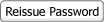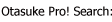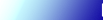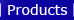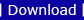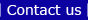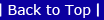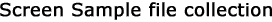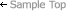Logic Sample Program>Logitouch Editor >Encode Process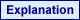DATA_D that has the bit positions (#n) in the conversion source register, DATA_S (integer 32-bit data) is created. An encode process can be actualized with much fewer steps than creating DATA_D with the Compare instruction (EQ) and the Movement instruction (M0V). If there's "1" in multiple bits, the encode process will be made with the higher bit.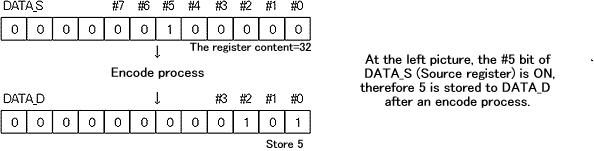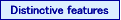In the case of conversion to values according to external input bit, if a circuit is created with the Compare/Movement formulas and the external input bit in a sequencer program, the Compare formula will increase by the number of input bits. Using the encode instruction above enables you to convert bits from 0 to 31 into numeric data with 2 rungs + (within Sub Routine) 3 rungs. Download a sample program file and detailed description.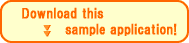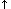Return to TopBack